# Find the Sum

Start with the basics! Build your math skills one at a time — or should we say, add them one at a time?Author
Taylor HartleyExpert Reviewer

Published: August 24, 2023# Find the Sum

Start with the basics! Build your math skills one at a time — or should we say, add them one at a time?Author
Taylor HartleyExpert Reviewer

Published: August 24, 2023# Find the Sum

Start with the basics! Build your math skills one at a time — or should we say, add them one at a time?Author
Taylor HartleyExpert Reviewer

Published: August 24, 2023Key takeaways

• Finding the sum is foundational – Addition is the most basic concept in math, so it’s important to master the skill before moving on.
• There are different ways to ask for and to find the sum – Someone might ask you to find the total or to combine something, and it’s up to you if you’d rather use your fingers or a number line!
• Practice makes perfect – Once you know how to find the sum, keep practicing to increase your speed and accuracy!

Finding the sum, or adding, is the most important yet basic concept in math. Without this skill, it will be almost impossible to move on to more complicated math concepts like subtracting, multiplying, and dividing. But, what is finding the sum?

It’s very simple — when we add two or more numbers together, the result is called the sum. Let’s dig in to learn more about addends and the different ways you may be asked to find the sum in the classroom and beyond.

## Let’s learn the termsJust beginning your math journey? Learning how to find the sum is a great place to start! One thing to keep in mind when it comes to math is that there’s a term or word to describe everything — a lot of it is memorization. For your first term, it’s important to know about addends.

Addends are a set of numbers that are being added together to find the sum. In other words, when we add two or more numbers, or addends, together, the answer we get is defined as the sum. Easy enough? Let’s take a look at a simple example.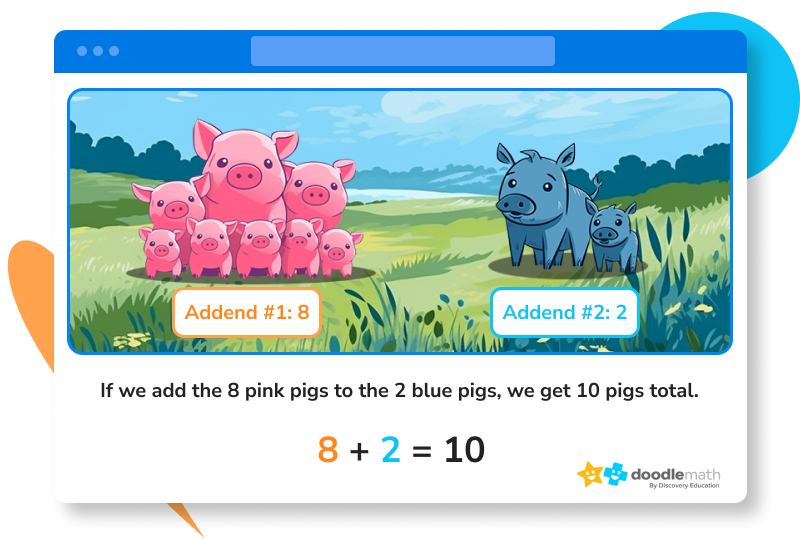## What is the sum?Simply put, the sum is a way of putting all of the things together. For those that might find math to be a bit tricky, let’s take it out of context for a second. Have you ever heard someone tell a story or explain a concept, then wrapped up by saying something like “To sum it up…” or “In summary…”? Both of these phrases come from the term “sum” and are used as ways to help recap or bring together all of the pieces of the puzzle.

Okay, back to math! Again, the sum helps us bring two or more numbers or addends together to make a total. Take a look at some other ways that you might be asked to find the sum:

• Put together….
• How many are there altogether?
• What is the total?
• What is the sum?
• How many are there combined?

You may see problems ask you for the sum like this: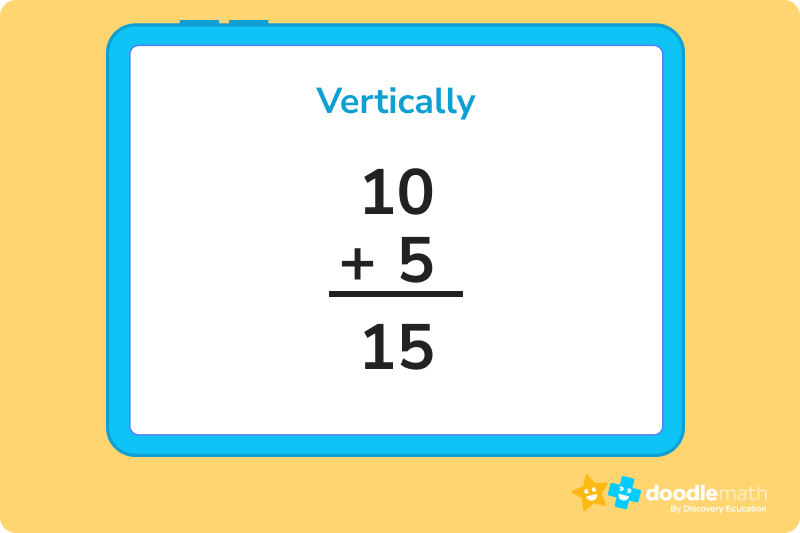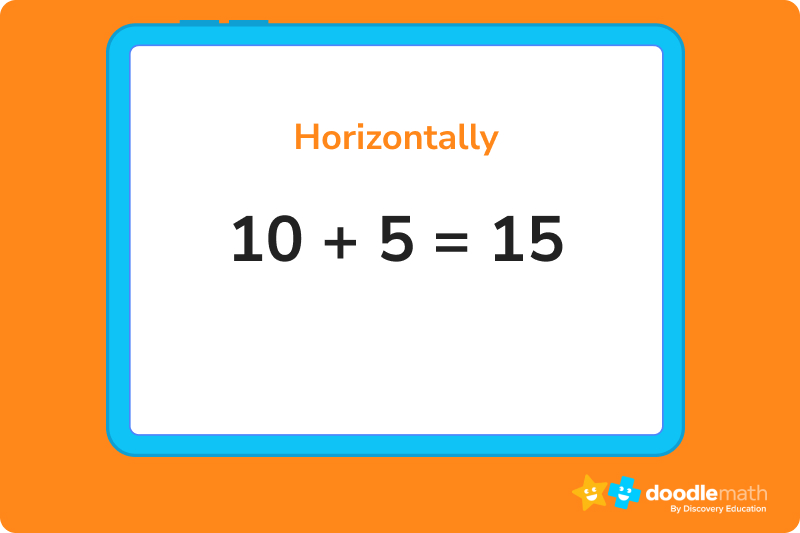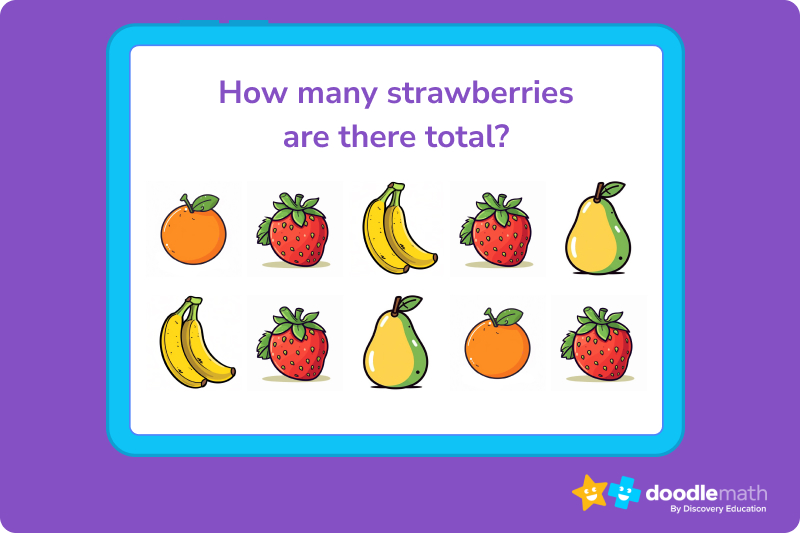## Finding the sum on a number lineFeel like using your fingers to find the sum? That’s totally fine! But, sometimes you may be asked to find the sum using a visual aid like a number line. To keep things simple, let’s imagine a number line from 0 to 10. Next, you’re asked to find the sum of 2 + 6.

First, find the 2 on the number line and mark it with your pencil. Then, since you need to add 6, you will hop 6 numbers down the line. An easy way to do this is to create little loops from one line to the next. Did you find the answer yet? Once you get six numbers down from 2, you end up on the number 8. Your final sum of 2 + 6 is 8!

Let’s try another example.

Let’s say we want to add 6 to 2. We will use our number line and start at the number 2.

Then, we hop 6 spaces, and we land on 8.

2 + 6 = 8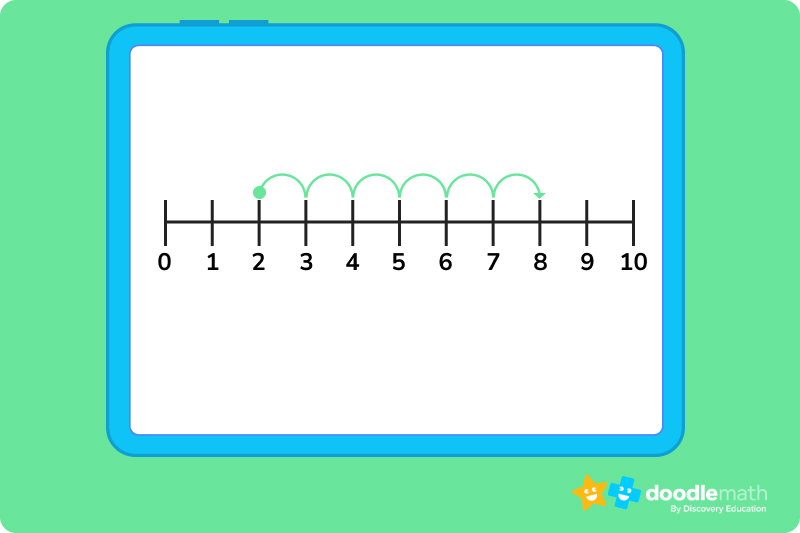Now you try!

What is 3+4?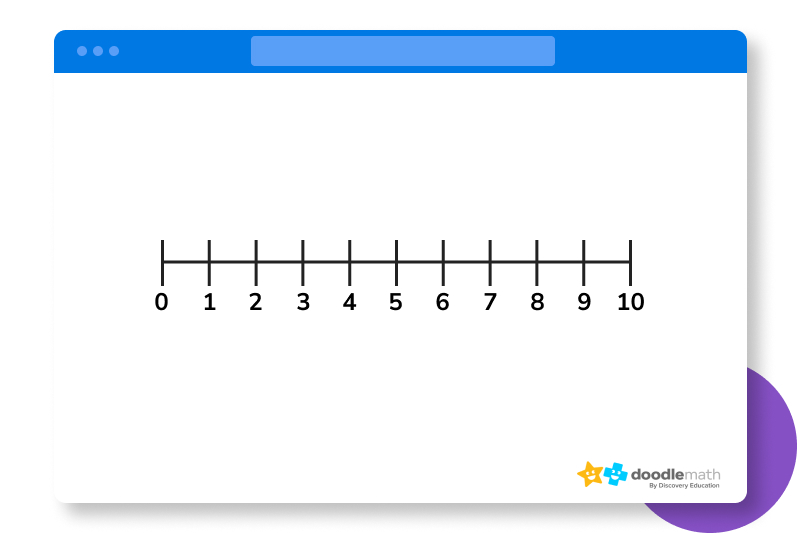## Practicing with sumsThe best way to learn how to find the sum is to practice! Below, let’s take a look at a few practice problems — each are asking you to find the sum, but they are worded differently. This way, you can get the hang of it while learning how you might be asked to find the sum in class or in more complex word problems!

1. What is the sum of 4 + 6? (Answer: 4 + 6 = 10)
2. Using a number line, what is 3 + 5? (Answer: 3 + 5 = 8)
3. If Jane has 6 apples and Billy has 8 apples, how many apples do Jane and Billy have in total? (Answer: 6 + 8 = 14 apples)

Ready to give it a go?

Now that we know how to find the sum, all of the ways that you may be asked to find the sum, and we’ve done several examples together using number lines, we’re ready to do a few more practice problems to put our new skills to the test! Work through the following problems on your own. Feel free to look back at the practice problems above if you get stuck, or if you need a quick reminder on how to find the sum.

And remember, don’t get discouraged if you run into a roadblock! Practice is the best way to learn something new.

## Parent Guide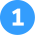How did we get here?

1. Read the problem: Using a number line, find the sum of 4 + 5.
2. Using a number line, locate the 4 and place your pencil on the line.
3. Then, make small loops along the way as you hop 5 spots to the right.
4. Your pencil will land on the number 9.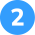How did we get here?

1. Read the problem: Jane and Josh want to make lemonade for their lemonade stand. Jane has 8 lemons and Josh has 9 lemons. How many lemons do they have combined?
2. Break the word problem down into a simple addition equation: 8 + 9
3. Now, use either a number line or simple counting to find the sum or 8 + 9
4. For this problem, we’ll use a number line. Locate the 8 and place your pencil on the line.
5. Then, make small loops along the way as you hop 9 spots to the right.
6. Your pencil will land on the number 17.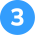How did we get here?

1. Break the addends up to avoid confusion. Start by adding 1 + 3 to get 4.
2. Then, add 6 to 4. Your new total is now 10.
4. Your final total is 18.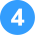C is incorrect.

How did we get here?

1. Read the problem: Which of the following is incorrect?
• 3 + 4 = 7
• 10 + 18 = 28
• 7 + 6 = 12
• 4 + 16 = 20
2. For each problem, find the sum of the two numbers to the left of the equal sign. You may use simple counting or a number line.
3. The answer to 3 + 4 = 7 which is correct.
4. The answer to 10 + 18 = 28 which is correct.
5. The answer to 7 + 6 = 13. The equation above shows 7 + 6 = 12 which is incorrect.
6. The answer to 4 + 16 = 20 which is correct.
7. Based on the answers you found to each equation, find the one that was incorrect.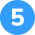How did we get here?

1. Read the problem: Fill in the blank: __ + 12 = 18
2. Using a number line, locate the 12 and place your pencil on the line.
3. Move your pencil up the line until you reach the number 18, counting how many spaces are in between 12 and 18.
4. There are 6 spaces, or hops, on the number line between 12 and 18.

## FAQs about math strategies for kidsWe understand that diving into new information can sometimes be overwhelming, and questions often arise. That’s why we’ve meticulously crafted these FAQs, based on real questions from students and parents. We’ve got you covered!

“Find the sum” is a mathematical phrase that refers to the process of adding numbers together to get a total. It involves combining or adding the given quantities to determine their collective value.

To find the sum of two or more numbers, you add them together. Start by writing down or mentally holding the numbers, then add each digit in the corresponding place value. Carry over any values that exceed the base value (such as carrying over from ones to tens). The result is the total sum of the given numbers.

Yes, the order in which you add the numbers does not affect the final sum. That’s because addition follows the commutative property, which means you can rearrange the numbers being added without changing the result. For example, when finding the sum of 3 + 4 + 5, you can add 3 + 5 first and then add 4, or vice versa, and you will still get the same sum.

Finding the sum is a fundamental skill used in various real-life situations. For example, it is used in calculating total prices when shopping, adding up scores in games or sports, determining the total distance traveled on a trip with multiple segments, or calculating the total number of items in a collection or group.## Related Posts

Title 1

Title 2

Title 3

Lesson credits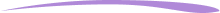Taylor Hartley

Taylor Hartley is an author and an English teacher based in Charlotte, North Carolina. When she's not writing, you can find her on the rowing machine or lost in a good novel.

Jill Padfield has 7 years of experience teaching high school mathematics, ranging from Alegra 1 to AP Calculas. She is currently working as a Business Analyst, working to improve services for Veterans while earning a masters degree in business administration.

Taylor Hartley

Taylor Hartley is an author and an English teacher based in Charlotte, North Carolina. When she's not writing, you can find her on the rowing machine or lost in a good novel.

Jill Padfield has 7 years of experience teaching high school mathematics, ranging from Alegra 1 to AP Calculas. She is currently working as a Business Analyst, working to improve services for Veterans while earning a masters degree in business administration.# Are you a parent, teacher or student?

Are you a parent or teacher?

## Hi there!

Book a chat with our team